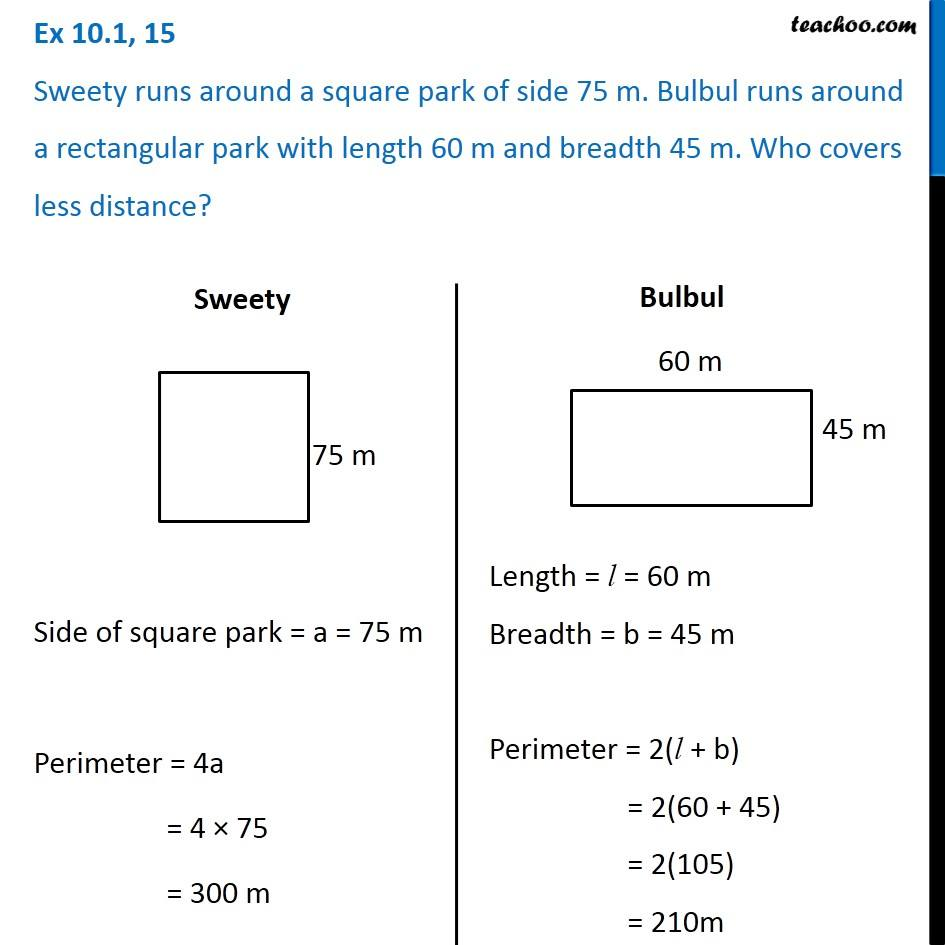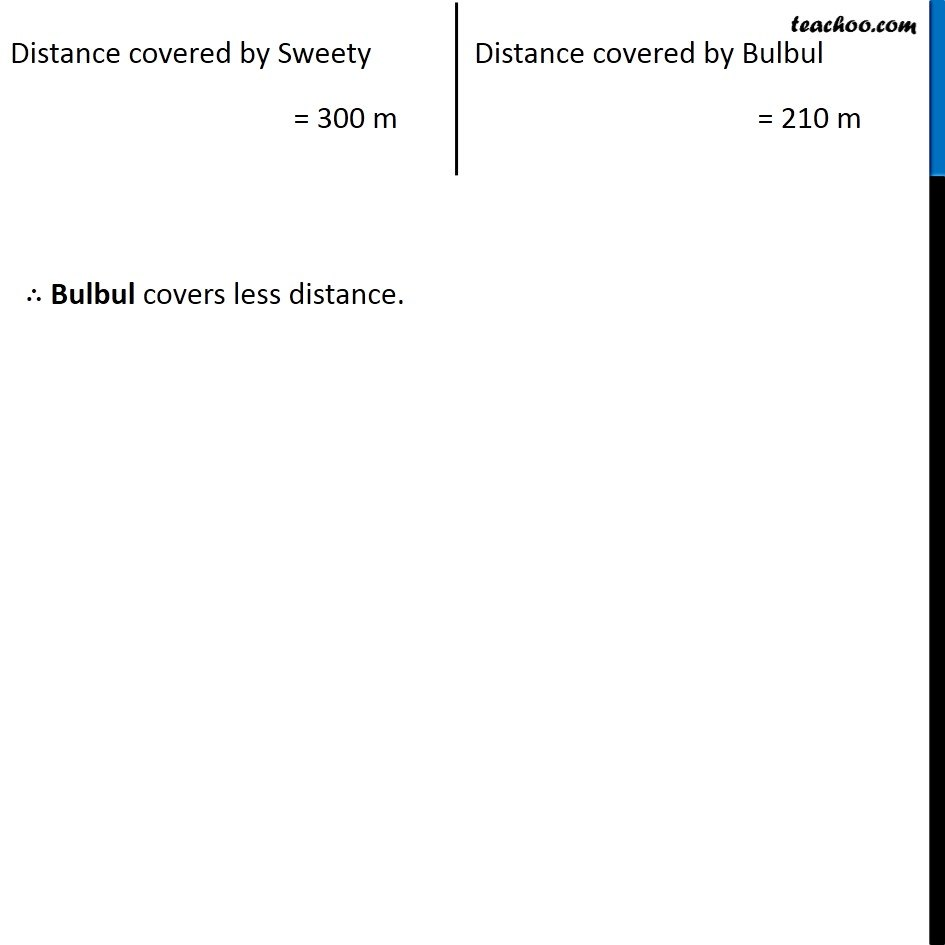Ex 10.1

Chapter 10 Class 6 Mensuration
Serial order wiseLearn in your speed, with individual attention - Teachoo Maths 1-on-1 Class

### Transcript

Ex 10.1, 15 Sweety runs around a square park of side 75 m. Bulbul runs around a rectangular park with length 60 m and breadth 45 m. Who covers less distance? Side of square park = a = 75 m Perimeter = 4a = 4 × 75 = 300 m Length = l = 60 m Breadth = b = 45 m Perimeter = 2(l + b) = 2(60 + 45) = 2(105) = 210m Distance covered by Sweety = 300 m Distance covered by Bulbul = 210 m ∴ Bulbul covers less distance.